Next: About this document ... Up: lab_template Previous: lab_template

Subsections

# Laplace, Heat, and Wave Equations

## Introduction

The purpose of this lab is to aquaint you with partial differential equations.

## Background

Second-order partial derivatives show up in many physical models such as heat, wave, or electrical potential equations. For example, the one-dimensional wave equation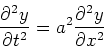can be solved by the displacement equation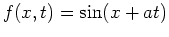, or, or even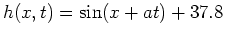. To show this, enter the displacement equation and then show that both sides of the differential equation are equal.
>f:=(x,t)->sin(x+a*t);
>g:=(x,t)->sin(k*x)*cos(k*a*t);
>h:=(x,t)->sin(x+a*t)+37.8;
>diff(f(x,t),t,t)-a^2*diff(f(x,t),x,x);
>diff(g(x,t),t,t)=a^2*diff(g(x,t),x,x);
>diff(h(x,t),t,t)=a^2*diff(h(x,t),x,x);


## Exercises

1. The function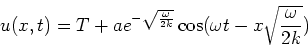denotes temperature at a depth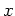and time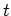where the seasonal variation of the surface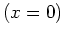temperature is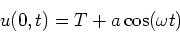Where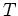is the annual average surface temperature and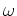is chosen such that the period is one year.
A)
Enter the function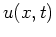B)
Show that the function satisfies the surface condition (i.e.).
C)
Show that the functionsatisfies the heat equation.
D)
Enter the following data into memory (Do this after completeing parts A, B, and C, or you will need to re-initialize the constants).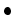The thermal conductivity of brick is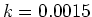.The average temperature of the surface temperature isdegrees celsius, please enter as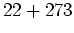as the conductivity uses units of Kelvin.The surface area of a brick is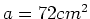E)
Plot the surface temperatureof a brick for one year (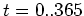). To make the temperature axis celsius, plot the function minus 273.
F)
From your graph, what is the approximate temperature variation of the surface of the brick?
G)
Plot the temperature of the inside of the brick with dimensions six by six by twelve (i.e. depth =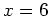). Remember to plot using celsius.
H)
From your graph, what is the temperature variation of the inside of the brick?
2. Determine which of the following functions satisfy Laplace's equationA)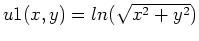B)C)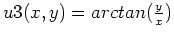D)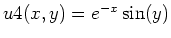3. Using a function from exercise 2 that you found satisfies the Laplace equation, answer the following without calculating the differential equation.
A)
Will the function plus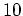satisfy the Laplace equation? Why or why not?
B)
Will the function plus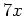satisfy the Laplace equation? Why or why not?
C)
Will the function plus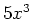satisfy the Laplace equation? Why or why not?Next: About this document ... Up: lab_template Previous: lab_template
Jane E Bouchard
2006-08-28